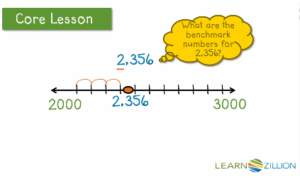In 4th grade students use strategies to estimate numbers. One strategy for estimation is rounding. This is built off of the foundation they received in 3rd grade. Below is how students learned how to use number lines to estimate numbers in 3rd grade.Five or more raise the score, four or less give it a rest! This saying and others similar to it are often used to help students remember how to round numbers. When these students are asked why they rounded 48 to 50, they explain that the 8 in the number 48 is five or more, so they raised the score. They are not able to explain that because 48 is only 2 away from 50, but is 8 away from 40, 48 should be rounded to 50. Open number lines can help students build conceptual understanding of how to round numbers.How to get students to use open number lines when rounding:
To build understanding of using open number lines to round, engage students with a human number line. Challenge students to round the number 76 to the nearest 10. Write the number 76 on an index card. Use a string (that 2 students hold) and have a student place the index card on the string with a clothes pin. Pose the question “What are the two closest tens to 76?” Students place the numbers 70 and 80 on either side of 76. Finding the mid-point between 70 and 80 will also help students know exactly where 76 should be on the number line. Ask students “Which ten is 76 closest to?” They will be able to see that 76 is closer to 80. They should also be able to explain that 76 is 4 away from 80.

After practice with a human number line, some students will be able to sketch an open number when rounding. Continue to expose students to this strategy when sequencing students while sharing their work.If you want to check out more about 3rd grade open number lines click the following link http://smathsmarts.com/rounding-number-lines/

Using an open number line to find benchmark numbers can help when rounding. If you have an example like the one below and were asked who is closer to the bakery? or who is closer to the pizza?This would be a easy visual and a way to prove your solution. Using that same idea we can use benchmark numbers to help us round on a number line. Below is an example of finding benchmark numbers for 2,356.If you are rounding to the nearest thousand you will get a much different solution than if you are rounding to the hundreds.We would use each of these strategies for different purposes. If you wanted just a ball park number you might want to round to the thousands place but if you wanted a more precise estimation, rounding to the hundreds place would be more appropriate. Either way, using a number line to estimate is a great tool.

To learn more about rounding to estimate using benchmark number click on the learnzillion lesson below. https://learnzillion.com/lesson_plans/6250